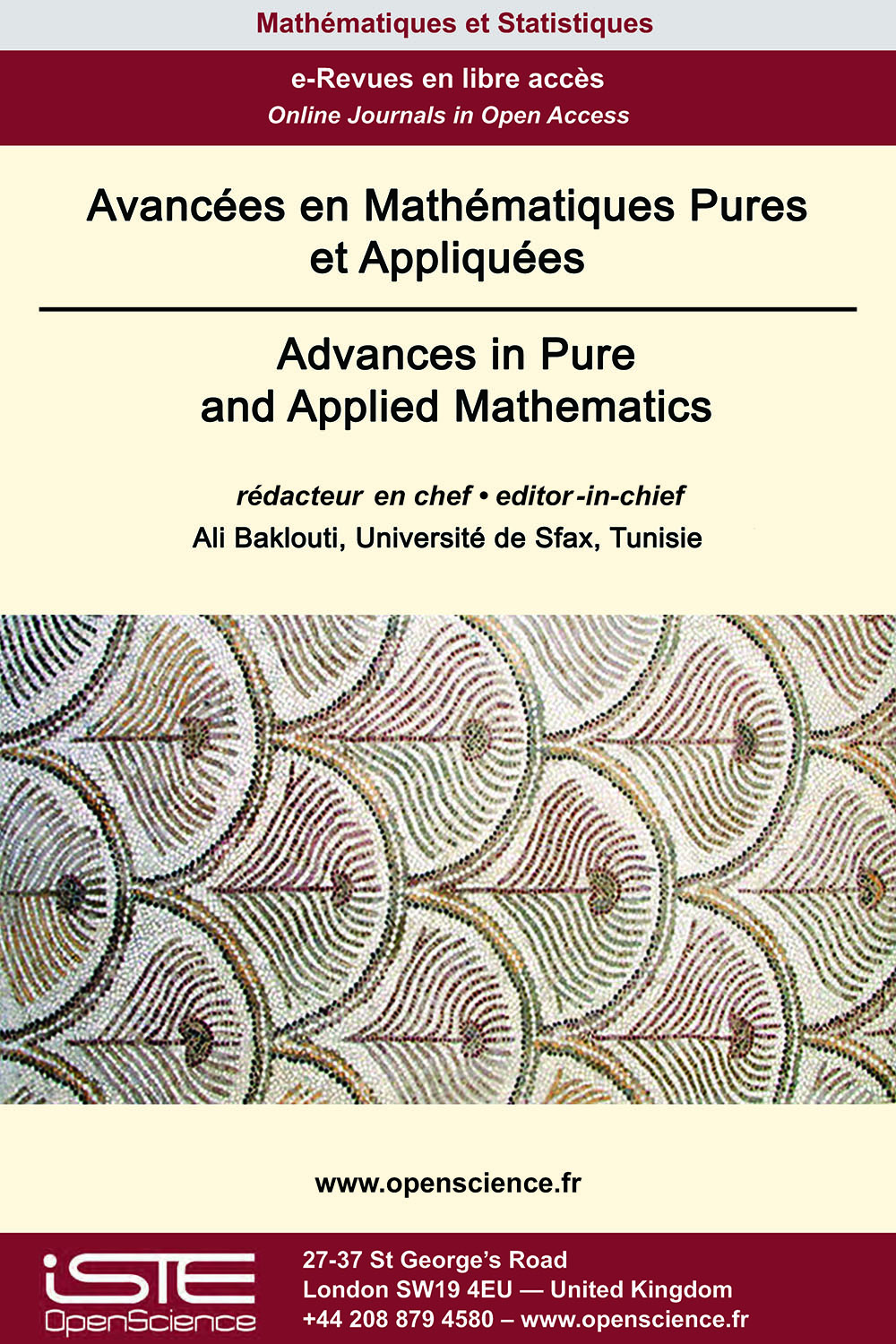# Vol 13 - À paraître

## Articles parus

[Forthcoming] Une nouvelle géneralisation des nombres de Genocchi et conséquence sur les polynômes de Bernoulli

This paper presents a new generalization of the Genocchi numbers and the Genocchi theorem. As consequences, we obtain some important families of integer-valued polynomials those are closely related to the Bernoulli polynomials. Denoting by ${(B_n)}_{n \in \mathbb{N}}$ the sequence of the Bernoulli numbers and by ${(B_n(X))}_{n \in \mathbb{N}}$ the sequence of the Bernoulli polynomials, we especially obtain that for any natural number $n$, the reciprocal polynomial of the polynomial $\big(B_n(X) - B_n\big)$ is integer-valued.

[Forthcoming] Estimations pondérées pour les opérateurs associés aux noyaux de Bergman-Besov

We characterize the weights for which we have the boundedness of standard weighted integral operators induced by the Bergman-Besov kernels acting between two weighted Lebesgue classes on the unit ball of ℂN in terms of Békollè - Bonami type condition on the weights. To accomplish this we employ the proof strategy originated by Békollè.

[Forthcoming] Transformation de Shearlet modulée continue

We generalize the well-known transforms such as short-time Fourier transform, wavelet transform and shearlet transform and refer it as Continuous Modulated Shearlet Transform. Important properties like Plancherel formula and inversion formula have been investigated. Uncertainty inequalities associated with this transform are presented.

[Forthcoming] Approches de points critiques pour les problèmes discrets anisotropes de type Kirchhoff

In this paper, we study a discrete anisotropic Kirchho type problem using variational methods and critical point theory, and we discuss the existence of two solutions for the problem. A example is presented to demonstrate the application of our main results.

[Forthcoming] Résultats d’existence pour l’equation du p(x)-laplacien singulier

This paper is concerned with the existence of solutions for the following class of singular fourth order elliptic equations
$\left\{ \begin{array}{ll} \Delta\Big(｜x｜^{p(x)}｜\Delta u｜^{p(x)-2}\Delta u\Big)=a(x)u^{-\gamma (x)}+\lambda f(x,u),\quad \mbox{in }\Omega, \\ u=\Delta u=0, \quad \mbox{on }\partial\Omega. \end{array} \right.$
where $\Omega$ is a smooth bounded domain in $\mathbb{R}^N, \gamma :\overline{\Omega}\rightarrow (0,1)$ be a continuous function, $f\in C^{1}( \overline{\Omega}\times \mathbb{R}), p:\; \overline{\Omega}\longrightarrow \;(1,\infty)$ and $a$ is a function that is almost everywhere positive in $\Omega$. Using variational techniques combined with the theory of the generalized Lebesgue-Sobolev spaces, we prove the existence at least one nontrivial weak solution.

[Forthcoming] Un résultat sur la Conjecture de Bruck sur les Polynômes Shift

This paper mainly concerns about establishing the Bruck conjecture for differential-difference polynomial generated by an entire function. The polynomial considered is of finite order and involves the entire function $f(z)$ and its shift $f(z + c)$ where $c \in ℂ$. Suitable examples are given to prove the sharpness of sharing exceptional values of Borel and Nevanlinna.

[Forthcoming] Ensembles limites et dynamique globale pour les 2-D champs de vecteurs sans divergence

The global structure of divergence-free vector fields on closed surfaces is investigated. We prove that if M is a closed surface and ${V}$ is a divergence-free $C^{1}$-vector field with finitely many singularities on M then every orbit L of $\mathcal{V}$ is one of the following types : (i) a singular point, (ii) a periodic orbit, (iii) a closed (non periodic) orbit in M* = M - Sing($\mathcal{V}$), (iv) a locally dense orbit, where Sing($\mathcal{V}$) denotes the set of singular points of $\mathcal{V}$. On the other hand, we show that the complementary in M of periodic components and minimal components is a compact invariant subset consisting of singularities and closed (non compact) orbits in M*. These results extend those of T. Ma and S. Wang in [Discrete Contin. Dynam. Systems, 7 (2001), 431-445] established when the divergence-free vector field $\mathcal{V}$ is regular that is all its singular points are non-degenerate.### Autres numéros :

2020

Volume 20- 11

Numéro 1 (Mai 2020)
Numéro 2 (Septembre 2020)

2021

Volume 21- 12

Numéro 1 (Janvier 2021)
Numéro 2 (Mai 2021)
Numéro spécial : AUS-ICMS 2020
Numéro 3 (Septembre 2021)

2022

Volume 22- 13

À paraître

Numéro 1 (Janvier 2022)
Numéro 2 (Mars 2022)